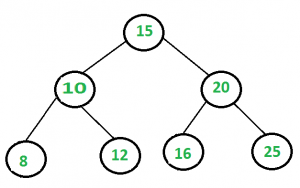# Find a pair with given sum in BST

Given a BST and a sum, find if there is a pair with given sum.

```Input : sum = 28
Root of below treeOutput : Pair is found (16, 12)
```

## Recommended: Please try your approach on {IDE} first, before moving on to the solution.

We have discussed different approaches to find a pair with given sum in below post.Find a pair with given sum in a Balanced BST

In this post, hashing based solution is discussed. We traverse binary search tree by inorder way and insert node’s value into a set. Also check for any node, difference between given sum and node’s value in set, if it is found then pair exists otherwise it doesn’t exist.

## C++

 `// CPP program to find a pair with ` `// given sum using hashing ` `#include ` `using` `namespace` `std; ` ` `  `struct` `Node { ` `    ``int` `data; ` `    ``struct` `Node *left, *right; ` `}; ` ` `  `Node* NewNode(``int` `data) ` `{ ` `    ``Node* temp = (Node*)``malloc``(``sizeof``(Node)); ` `    ``temp->data = data; ` `    ``temp->left = NULL; ` `    ``temp->right = NULL; ` `    ``return` `temp; ` `} ` ` `  `Node* insert(Node* root, ``int` `key) ` `{ ` `    ``if` `(root == NULL) ` `        ``return` `NewNode(key); ` `    ``if` `(key < root->data) ` `        ``root->left = insert(root->left, key); ` `    ``else` `        ``root->right = insert(root->right, key); ` `    ``return` `root; ` `} ` ` `  `bool` `findpairUtil(Node* root, ``int` `sum,  unordered_set<``int``> &set) ` `{ ` `    ``if` `(root == NULL) ` `        ``return` `false``; ` ` `  `    ``if` `(findpairUtil(root->left, sum, set)) ` `        ``return` `true``; ` ` `  `    ``if` `(set.find(sum - root->data) != set.end()) { ` `        ``cout << ``"Pair is found ("` `             ``<< sum - root->data << ``", "` `             ``<< root->data << ``")"` `<< endl; ` `        ``return` `true``; ` `    ``} ` `    ``else` `        ``set.insert(root->data); ` ` `  `    ``return` `findpairUtil(root->right, sum, set); ` `} ` ` `  `void` `findPair(Node* root, ``int` `sum) ` `{ ` `    ``unordered_set<``int``> set; ` `    ``if` `(!findpairUtil(root, sum, set)) ` `        ``cout << ``"Pairs do not exit"` `<< endl; ` `} ` ` `  `// Driver code ` `int` `main() ` `{ ` `    ``Node* root = NULL; ` `    ``root = insert(root, 15); ` `    ``root = insert(root, 10); ` `    ``root = insert(root, 20); ` `    ``root = insert(root, 8); ` `    ``root = insert(root, 12); ` `    ``root = insert(root, 16); ` `    ``root = insert(root, 25); ` `    ``root = insert(root, 10); ` ` `  `    ``int` `sum = 33; ` `    ``findPair(root, sum); ` ` `  `    ``return` `0; ` `} `

## Python3

 `# Python3 program to find a pair with  ` `# given sum using hashing  ` `import` `sys ` `import` `math ` ` `  `class` `Node: ` `    ``def` `__init__(``self``,data): ` `        ``self``.data ``=` `data ` `        ``self``.left ``=` `None` `        ``self``.right ``=` `None` ` `  `def` `insert(root,data): ` `    ``if` `root ``is` `None``: ` `        ``return` `Node(data) ` `    ``if``(data < root.data): ` `        ``root.left ``=` `insert(root.left, data) ` `    ``if``(data > root.data): ` `        ``root.right ``=` `insert(root.right, data) ` `    ``return` `root ` ` `  `def` `findPairUtil(root, summ, unsorted_set): ` `    ``if` `root ``is` `None``: ` `        ``return` `False` `    ``if` `findPairUtil(root.left,summ,unsorted_set): ` `        ``return` `True` `    ``if` `unsorted_set ``and` `(summ``-``root.data) ``in` `unsorted_set: ` `        ``print``(``"Pair is Found ({},{})"``.``format``(summ``-``root.data, root.data)) ` `        ``return` `True` `    ``else``: ` `        ``unsorted_set.add(root.data) ` ` `  `    ``return` `findPairUtil(root.right,summ, unsorted_set) ` ` `  `def` `findPair(root,summ): ` `    ``unsorted_set ``=` `set``() ` `    ``if``(``not` `findPairUtil(root,summ,unsorted_set)): ` `        ``print``(``"Pair do not exist!"``) ` ` `  `# Driver code ` `if` `__name__``=``=``'__main__'``: ` `    ``root``=``None` `    ``root ``=` `insert(root,``15``) ` `    ``root ``=` `insert(root,``10``) ` `    ``root ``=` `insert(root,``20``) ` `    ``root ``=` `insert(root,``8``) ` `    ``root ``=` `insert(root,``12``) ` `    ``root ``=` `insert(root,``16``) ` `    ``root ``=` `insert(root,``25``) ` `    ``root ``=` `insert(root,``10``) ` `    ``summ ``=` `33` `    ``findPair(root, summ) ` ` `  `# This code is contributed by Vikash Kumar 37 `

Output:

```Pair is found (8, 25)
```

Time Complexity is O(n).

My Personal Notes arrow_drop_upCheck out this Author's contributed articles.

If you like GeeksforGeeks and would like to contribute, you can also write an article using contribute.geeksforgeeks.org or mail your article to contribute@geeksforgeeks.org. See your article appearing on the GeeksforGeeks main page and help other Geeks.

Please Improve this article if you find anything incorrect by clicking on the "Improve Article" button below.

Improved By : Vikash Kumar 37